# ICDAR2013文本检测算法的衡量方法（二）Rectangle Matching与DetEvalOCR 同时被 2 个专栏收录6 篇文章 0 订阅

• Rectangle Matching， 或者bbox matching
• ICDAR2013 Foucsed Image 文本检测任务的evaluation方法

• evaluation/衡量方法都是只针对text localization算法；
• text detection 与 text localization 不作区分
• bounding box, bbox, rectangle不作区分

# Detection with bbox

1. pixel feature discriminace
2. pixel classification
3. detection at rectangle
4. target oriented

# evaluation问题描述

Evaluation问题的输入：

1. D $D$, 要衡量的检测算法输出的bbox集合.
2. G$G$, Ground Truth bbox集合.
3. Di,Gj $D_i, G_j$分别代表 D,G $D, G$ 的一个元素
输出： 对 D $D$的质量评估。

# Matching of two bboxes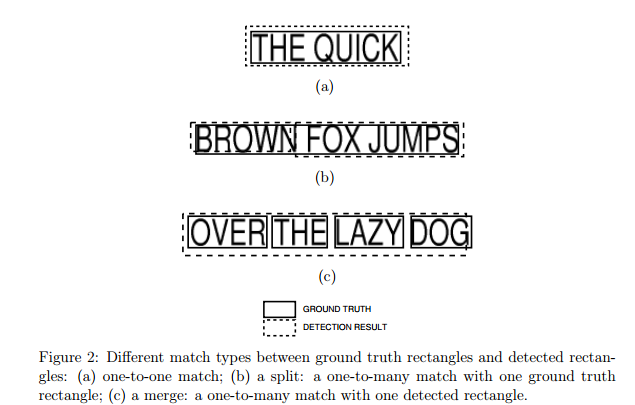1. 一对一的match，如（a）所示。
2. 一对多的match，如（b）所示，ground truth 粒度大于detection 粒度时出现的情况。
3. 多对一的match，如（c）所示，detection的粒度大于ground truth的粒度。
4. 多对多的match，图中没有画出。
不同的evaluation方法的不同的根源就在于对这三种match的处理方式不同

# Evaluation in ICDAR2003，只考虑一对一的match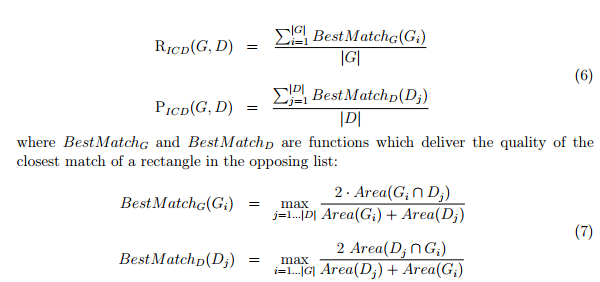（7）中 BestMatch $BestMatch$的分式计算形式看起来跟F-mean很相似。实际上它就是一个F-mean/调和平均数（Harmonic Mean）。可以定义基于面积的、bbox适用的recall 与precision：

R(Gi,Dj)=Area(GiDj)Area(Gi)

P(Gi,Dj)=Area(GiDj)Area(Dj)

MatchScore(Gi,Dj)=2R(Gi,Dj)P(Gi,Dj)R(Gi,Dj)+P(Gi,Dj)=2Area(GiDj)Area(Gi)+Area(Dj)

BestMatch(Gi,D)=maxjMatchScore(Gi,Dj)

# DetEval——Evaluation in ICDAR2013，考虑了三种match方式

ICDAR2013则使用了新evaluation方法：DetEval，也就是十几年前Wolf提出的方法。“新方法”同时考虑了一对一，一对多，多对一的情况， 但不能处理多对多的情况。（作者说，实验结果表示在文本检测里这种情况出现的不多。）

## 计算recall matrix与precision matrix

recall 与precision两个矩阵分别用 σ $\sigma$ τ $\tau$表示，它们都是 |G|×|D| $|G| \times |D|$的矩阵，每一行代表一个 Gi $G_i$, 每一列代表一个 Dj $D_j$:

σij=R(Gi,Dj)

τij=P(Gi,Dj)

## 利用 σ,τ $\sigma,\tau$表示三种match

tr,tp[0,1] $t_r, t_p \in[0,1]$分别为 σ,τ $\sigma, \tau$的阈值。算法能表示除了many-to-many以外的三种match方式：

• one-to-one: 一个 Gi $G_i$只与一个 Dj $D_j$匹配
• Gi $G_i$的大部分范围只被 Dj $D_j$检测出来： σ $\sigma$的第 i $i$行只有σijtr$\sigma_{ij} \ge t_r$
• 只有 Dj $D_j$检测 Gi $G_i$的precision符合要求: τ $\tau$的第 j $j$列只有τijtp$\tau_{ij} \ge t_p$
• one-to-many（splits）: Gi $G_i$ D $D$的一个元素数量大于1的子集Do$D_o$检测出来：
• Do $D_o$中的每个元素都能一定准确度检测出 Gi $G_i$的一部分： τijtp,jDo $\tau_{ij} \ge t_p, \forall j \in D_o$
• Gi $G_i$的大部分范围被 So $S_o$检测出来： jSoσijtr $\sum_{j \in S_o} \sigma_{ij} \ge t_r$
• many-to-one(merges) : G $G$一个元素个数大于1的子集Go$G_o$被一个检测结果 Dj $D_j$检测出来
• Go $G_o$中的每个元素都有一定比例的面积被检测出来： σijtr,iGo $\sigma_{ij} \ge t_r, \forall i \in G_o$
• Dj $D_j$ 以一定准确度检测出 Go $G_o$ iGoτijtp $\sum_{i \in G_o} \tau_{ij} \ge t_p$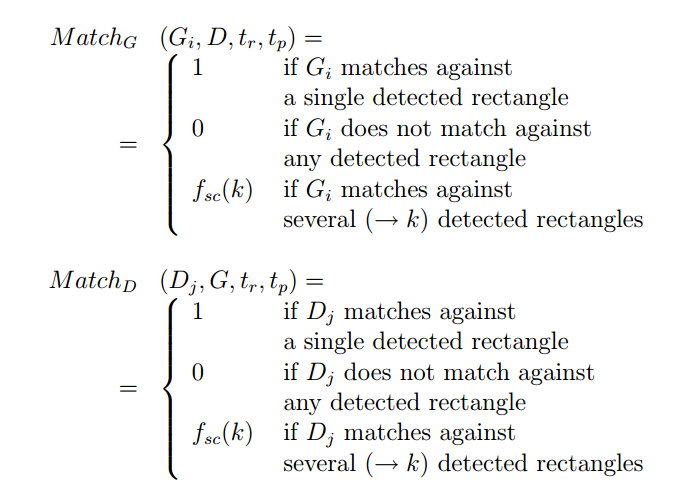1. 不惩罚one-one match。只要匹配的recall与precision都大于各自的阈值就行。它与ICDAR2003又有不同：ICDAR2003的考虑one-one match时是以soft方式计算recall与precision，不取阈值，所以ICDAR2003虽然只考虑one-one match，但根据match的好坏会有不同的惩罚。
2. fsc(k) $f_{sc}(k)$中的 k $k$是many-to-one或one-to-many里many的具体数值, 下标sc代表scatter。fsc(k)$f_{sc}(k)$可以是一个0到1之间的常数（作者的做法），也可以是一个关于 k $k$的函数， 例如fsc=11+ln(k)$f_{sc} = \frac{1}{1 + ln(k)}$

## 计算单张图片与多张图片上的recall 与precision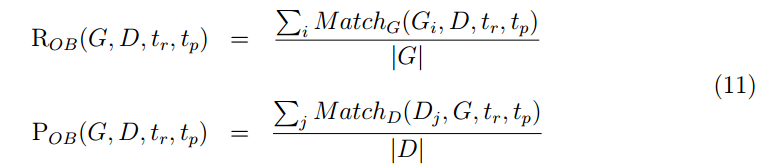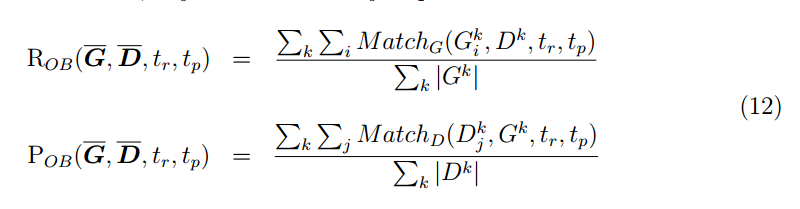## 最终的recall 与precision

evalfixed det.xml gt.xml

* 以 tr,tp $t_r, t_p$ x,y $x, y$轴， recall/precision $recall/precision$ z $z$轴， 可以得到两个3D的函数图像。 固定tr,tp$t_r, t_p$中的一个， 可以得到2D函数图像。作者说它们可以用于更深入的分析算法性能， 但个人觉得并没有提供太多参考信息。
* 最终的recall与precision是采用与mAP类似计算方式：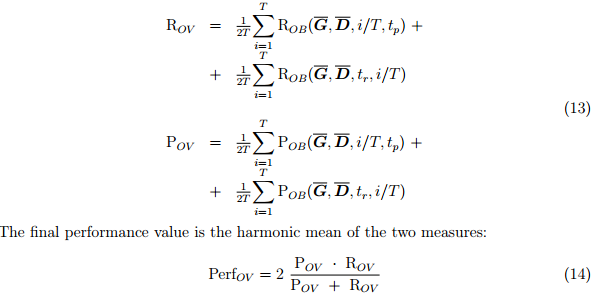# ICDAR 2013与Deteval

• ICDAR2013 Task1的evaluation页面提供了ICDAR2013Deteval两种evaluation选择。前者是ICDAR根据Deteval 论文实现的代码，与paper作者的实现有细微差别，具体见http://rrc.cvc.uab.es/?ch=2&com=faq
• 它们使用了相同的参数 tr=0.8,tp=0.4 $t_r = 0.8, t_p = 0.4$，这样做的目的是为了加大对只检测出一部分文本区域的结果的惩罚，因为这种检测结果无法识别。
• 因为ICDAR2013的ground truth已经是word level的，所以衡量算法对one-to-many（检测结果将一个word分成了好几个bbox）的惩罚要大于many-to-one（将多个word用一个bbox框出来）。事实上，对后者没有惩罚。即： fsc(k)=0.8 for one-to-many, fsc(k)=1 for many-to-one $f_{sc}(k) = 0.8 \text{ for one-to-many, }f_{sc}(k) = 1 \text{ for many-to-one}$
这一点还是很合理的。
08-11
12-0670108-011380
09-231784
07-095795
12-2052
05-108647
10-31
01-04
05-25
12-161万+
03-131392
09-121万+
01-142200
05-096178
06-144079点击重新获取扫码支付余额充值